# A brief introduction to deep neural networks.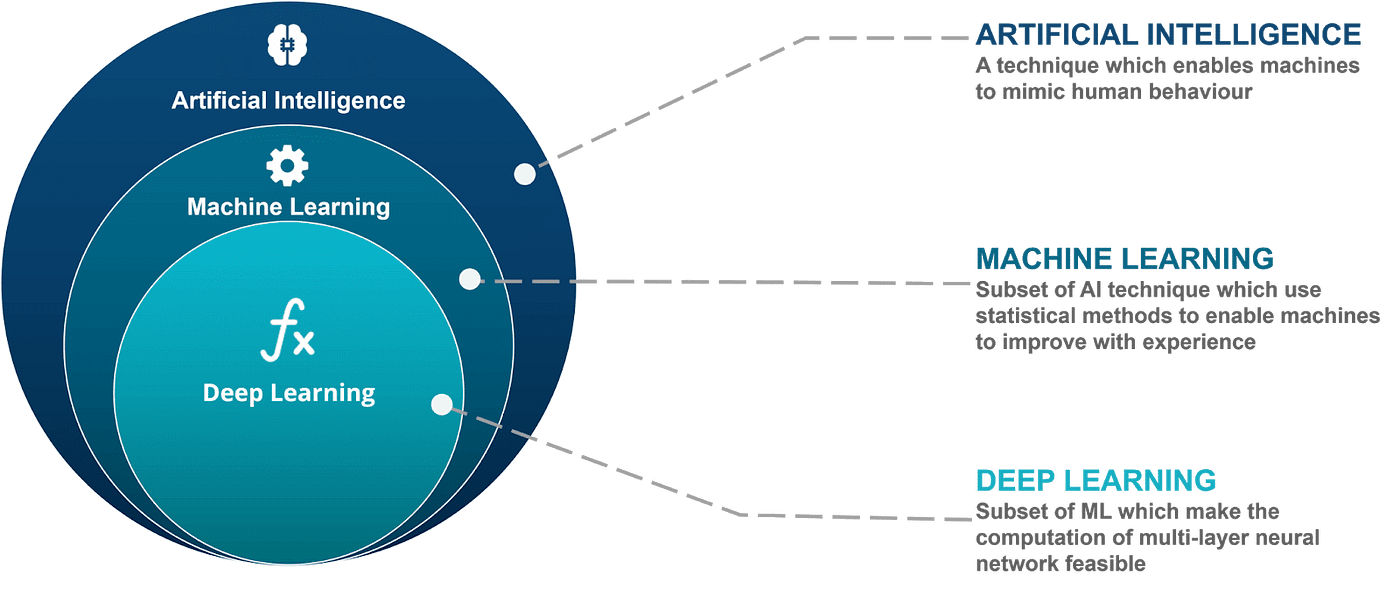A picture of the subsets of AI, with Deep Learning being a subset of ML. Image from https://mrrajeshrai.com/artificial-intelligence-vs-machine-learning-vs-deep-learning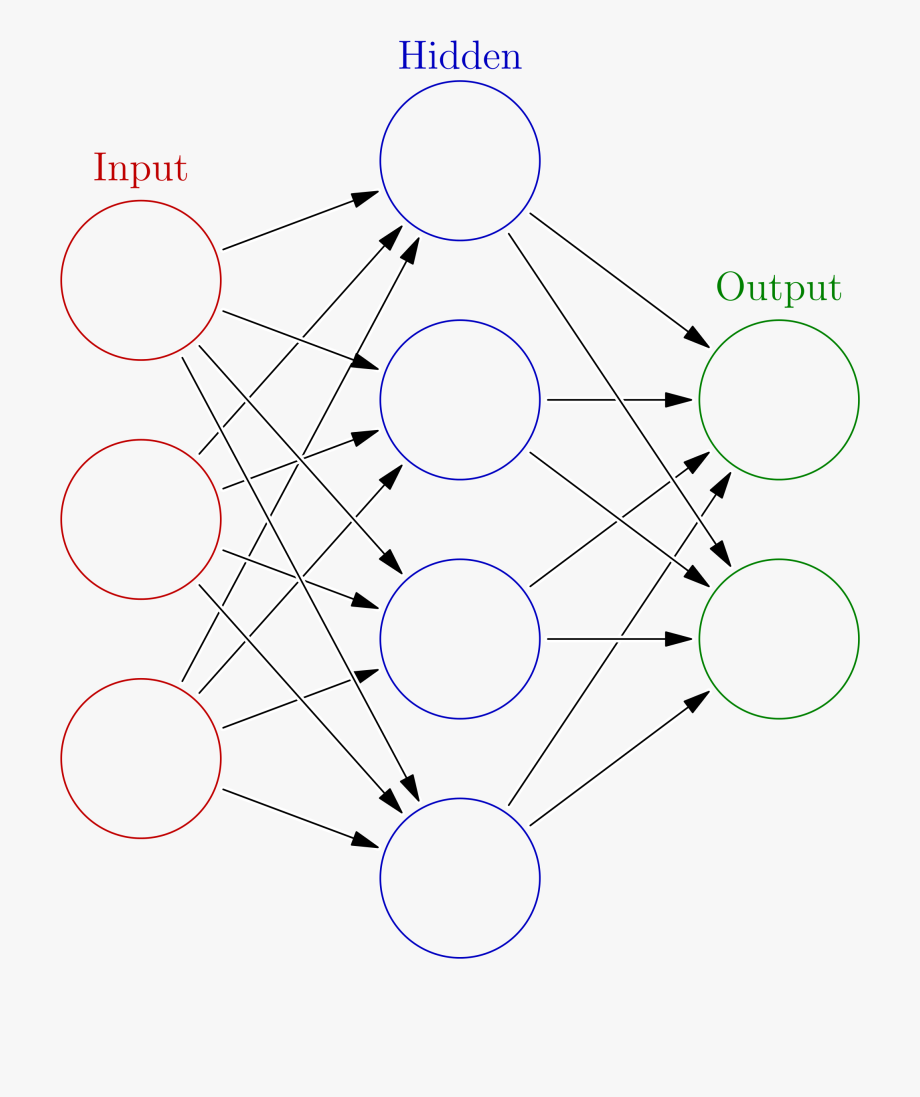A picture of what a neural network looks like. Image from https://www.clipartwiki.com/iclip/wTJxmh_filecolored-neural-network-simple-neural-network/

# The main framework for neural networks

In very simple terms, let’s say we are trying to plot 1 red point and 1 blue point inside of a 2D graph. The one thing the ANN will try to do is find the line that separates them the best. The way the algorithm does this is by determining how far the line is from the point, and the line either moves farther away or closer to it, depending on whether it’s classified properly or not. Behind the scenes, the weights and biases for all the nodes are changing because of this.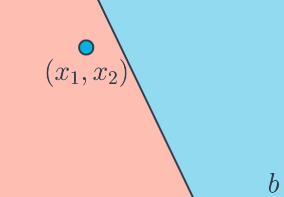The blue point in this picture is not classified properly, so it wants the line to move towards it to classify it. This is essentially what a neural network does.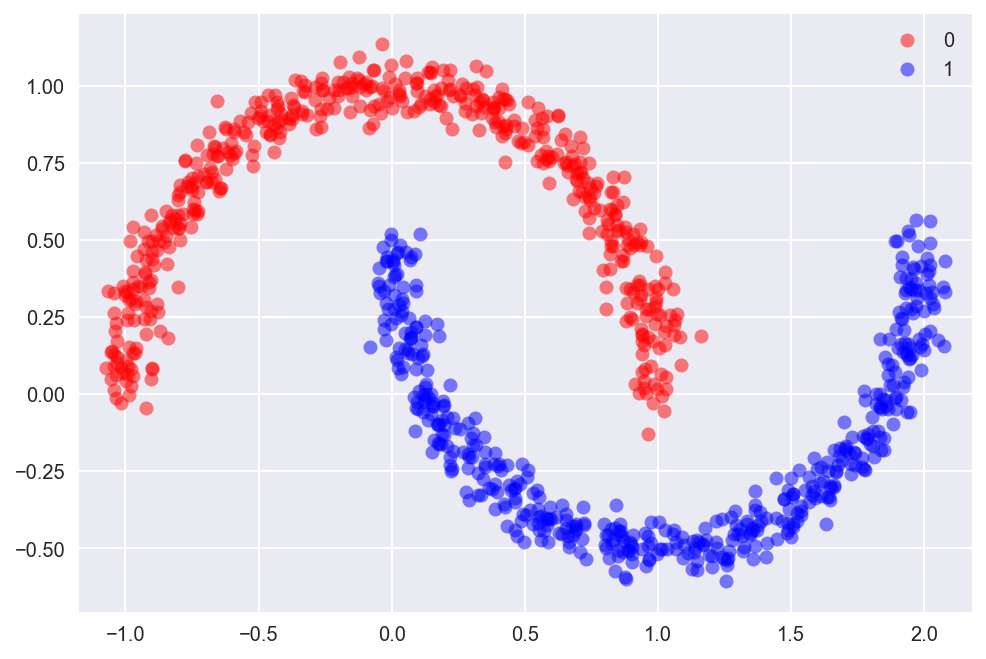A more complex dataset. Not something you would see often in real life but the same techniques can be applied for classifying the ANN here as any other.

# Activation Functions

Now, let’s take a deeper look into activation functions. The activation function is used to put the range of the output between a certain range for all of the node outputs. They are the ones that convert the output from one layer into the input for the next layer. There are many activation functions, so let’s take a closer look at a few notable ones.

## The Sigmoid Function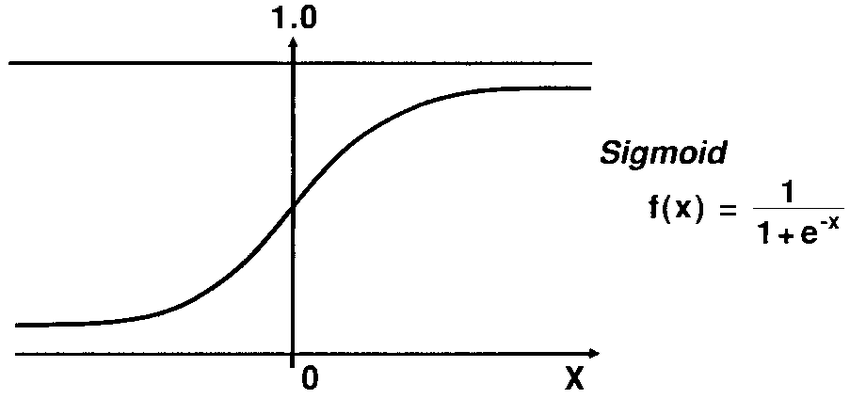A picture of the sigmoid function. Picture from https://www.researchgate.net/figure/An-illustration-of-the-signal-processing-in-a-sigmoid-function_fig2_239269767

## The Hyperbolic Tangent Function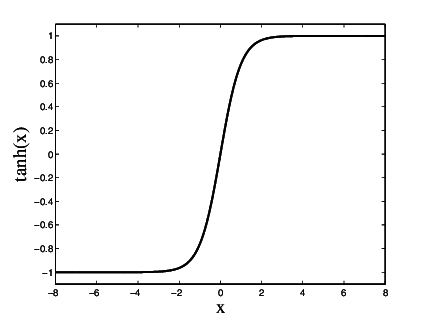A picture of the hyperbolic tangent function. Image from https://www.researchgate.net/figure/The-Hyperbolic-Tangent-Activation-Function_fig1_224535485

## Rectified Linear Unit Activation Function (ReLU)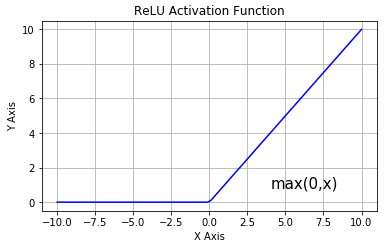a picture of the Rectified linear unit function mapping. Image from https://www.researchgate.net/figure/Activation-function-Rectified-Linear-Unit-ReLU_fig1_328905193

## Softmax Function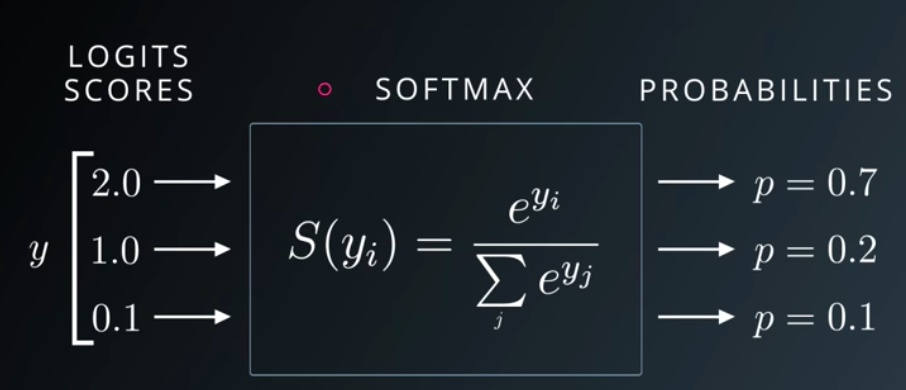A picture of the softmax function returning probabilities to the
• Weights, biases and nodes
• Multi-layer neural networks
• Activation functions